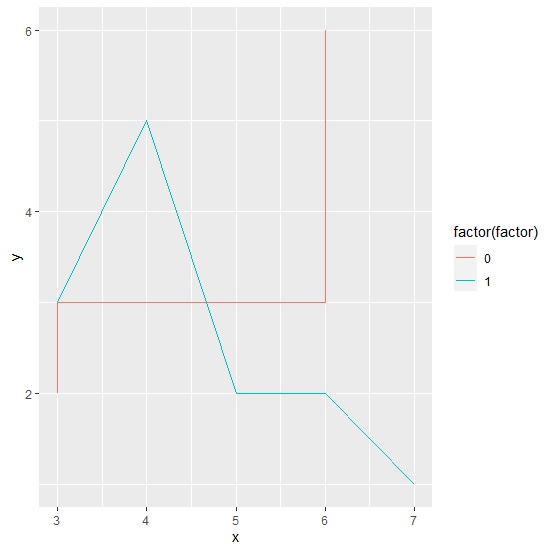# How to create two lines using ggplot2 based on a categorical column in R?

To create two lines using ggplot2 based on a categorical column in R, we can follow the below steps −

• First of all, create a data frame.
• Then, create the line chart with categorical column read as factor inside aes of ggplot function.

## Create the data frame

Let's create a data frame as shown below −

Live Demo

x<-rpois(10,5)
y<-rpois(10,2)
factor<-sample(0:1,10,replace=TRUE)
df<-data.frame(x,y,factor)
df

On executing, the above script generates the below output(this output will vary on your system due to randomization) −

x y factor
1 3 2 0
2 7 1 1
3 3 3 0
4 3 3 1
5 6 2 1
6 5 2 1
7 5 3 0
8 4 5 1
9 6 3 0
10 6 6 0

## Create lines using ggplot2 based on categorical column

Loading ggplot2 package and creating line chart based on values in factor column of df −

x<-rpois(10,5)
y<-rpois(10,2)
factor<-sample(0:1,10,replace=TRUE)
df<-data.frame(x,y,factor)
library(ggplot2)
ggplot(df,aes(x,y,color=factor(factor)))+geom_line()

### Output